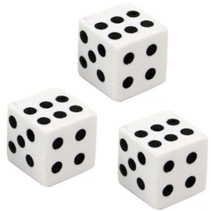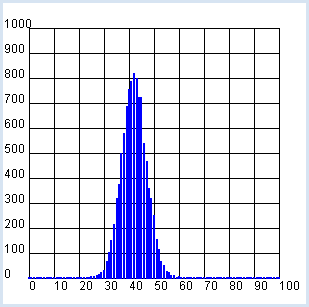deutsch     english    français     Print

## 8.1 SIMULATIONS### INTRODUCTION

 Computer simulations do not only play an important role in research and in the industry, but also also in the finance world. They are used to simulate the behavior of a real system using a computer. Computer simulations have the advantage of being inexpensive and environmentally friendly, as well as safe, compared to real experiments and studies. However, they can usually never reflect reality with full accuracy. There are many reasons for this: reality can never be perfectly represented by numbers due to errors in measurement (except for in enumerations) often the interaction of the components is not precisely known, since either the underlying laws are not exact [more...Most natural laws provide for quantum phenomena anyway only statistical statements] or not all influences are taken into account [more... The number of components involved in many-body systems can be of the order of 1023 ] Nevertheless, computer simulations are becoming more and more precise with increasing computational power, just think of the weather forecasts for the next few days. Chance plays an exceptionally big role in our lives, as we make many decisions based on an intuitive assessment of probabilities and not just on the basis of purely logical arguments. However, the use of chance can also greatly simplify problems with exact solutions. One example is that it can be very time consuming to exactly determine the shortest possible path from A to B on a road map with many different connection possibilities using an algorithm; it is sufficient for practical use to find the most probably shortest possible path [more...These are randomized algorithms, a recent research area of great importance] PROGRAMMING CONCEPTS: Computer simulation. computer experiment, statistical fluctuations

### THE COMPUTER AS A GAME PARTNER

 Your companion Nora suggests the following game: "You can throw three dice. If you roll a six, you win and I'll give you a marble. If you don't roll a six, I win and you have to give me a marble". At first glance the game appears to be fair because you think about it quickly and realize that for each die, the probability of rolling a six is 1/6, and so the chance to roll a six in the first, second, or third turn is 1/6 + 1/6 + 1/6 = 1/2.You can verify this thought process with the computer and your programming skills. You thereby assume that it does not matter whether you roll the 3 dice consecutively or all at the same time. So in other words, the probability of a die to obtain a certain number is independent of the other dice and it is always 1/6.
There are two ways to tackle the problem, either statistically or combinatorially. The statistical solution corresponds to the real game. You simulate the throwing of the dice by repeatedly generating 3 random numbers between 1 and 6 and then counting the winning cases.

```from random import randint

n = 1000 # number of games
won = 0
repeat n:
a = randint(1, 6)
b = randint(1, 6)
c = randint(1, 6)
if a == 6 or b == 6 or c == 6:
won += 1

print("Won:", won, " of ", n, "games")
print("My winning percentage:", won / n)```
Highlight program code (Ctrl+C copy, Ctrl+V paste)

The result is a winning percentage of about 0.42, not 0.5 as you expected. The value easily changes from simulation to simulation though, because it is subject to statistical fluctuations. As you might intuitively expect, the result is more accurate the more tests you do.

Statistical fluctuations are of great importance in computer simulations.

 In order to examine them, you conduct the experiment with purposely few games (let's say 100) many times (let's say 10000 times) and draw a frequency diagram of the games won. It results in an interesting bell-shaped distribution, typical for statistics. You use a GPanel as a graphics window in the program. You can also display a coordinate grid using drawGrid(). Implement a single hundred-times test with the function sim(), which returns the number of games won whose fluctuations you want to investigate.```from gpanel import *
from random import randint

z = 10000
n = 100

def sim():
won = 0
repeat n:
a = randint(1, 6)
b = randint(1, 6)
c = randint(1, 6)
if a == 6 or b == 6 or c == 6:
won += 1
return won

makeGPanel(-10, 110, -100, 1100)
drawGrid(0, 100, 0, 1000)
h =  * (n + 1)
repeat z:
x = sim()
h[x] += 1
title("Simulation ended")

lineWidth(2)
setColor("blue")
for x in range(n + 1):
line(x, 0, x, h[x])
```
Highlight program code (Ctrl+C copy, Ctrl+V paste)

### MEMO

 The maximum of the distribution is approximately at 42, since the probability of winning is around 0.42 and you play 100 games each time. If you play 100 times with Nora it is possible that you win the game over 50 times, despite your only 0.42 chance of winning. However, the probability for this is quite low (ca. 5 %) and therefore the game is not fair. Computer experiments with random numbers are subject to statistical fluctuations that get smaller the larger number of attempts.

For the combinatorial solution, you let the computer run all possible rolls with 3 dice one after another. The first, second, and third roll can each result in a number from 1 to 6. In the nested for loop you form all triples of numbers and count the total possibilities with the variable possible, whereas you count your winning cases which contain at least one 6 with the variable favorable.

```possible = 0
favorable = 0
for i in range(1, 7):
for j in range(1, 7):
for k in range(1, 7):
possible += 1
if i == 6 or j == 6 or k == 6:
favorable += 1
print("favorable:", favorable, "possible:", possible)
print("My winning percentage:", favorable / possible)```
Highlight program code (Ctrl+C copy, Ctrl+V paste)

This results in 91 favorable of 216 possible cases and thus a winning probability w = favorable / possible of 91/216 = 0.42, which you also get with the computer simulation.

You could, of course, also solve this problem entirely without a computer. For this, think of the following: There are three possible winning events E1, E2, E3:

 E1: Getting a 6 on the first roll. Probability: 1/6 E2: No 6 in the first roll, but a 6 in the second round. Probability: 5 /6 * 1/6 E3: No 6 in either the first or second roll, but a 6 in the third round. Probability: 5/6 * 5 /6 * 1/6

Since E1, E2, and E3 are independent of each other, the probability is the sum, i.e. 1 /6 + 5 /36 + 25 /216 = 91/216 = 0.421296.

You can display the process as a tree:There is also an ideal way to get the solution: the probability of rolling no 6's at all is p = 5/6 * 5/6 * 5/6 = 125/216. Therefore, the desired probability is w = 1 - p = 91/216.

### EXERCISES

1.

The Duke Ferdinando de Medici of Florenz determined in the year 1600 that when throwing 3 dice a total of 9 or 10 pips (the dots on the die) can be obtained with a same number of possibilities:

 Augensumme 9 Augensumme 10 1 + 2 + 6 1 + 3 + 6 1 + 3 + 5 1 + 4 + 5 1 + 4 + 4 2 + 2 + 6 2 + 2 + 5 2 + 3 + 5 2 + 3 + 4 2 + 4 + 4 3 + 3 + 3 3 + 3 + 4

The Duke found, however, that rolling the totals of  9 and 10 are not equally probable  and he asked mathematics professor Galileo Galilei for advice. Calculate these probabilities with a computer simulation in two ways:
a. statistically
b. combinatorially

2.

Using a statistical computer simulation, determine the probability that at least two children have the same birthday (no leap year) in a class of (at least) 20 children.

3.

In 1650 in Paris, the Chevalier de Méré asked the mathematician Blaise Pascal about the odds of the following two events:
a. rolling at least one 6 after 4 rolls
b. rolling at least one double 6 after 24 rolls.
He believed that the odds of winning are equal, since even though with b) the probability of winning is 6 times as low, there 6 times as many tries. Was he right?

4*.

In the game with Nora, determine how high the probability is that you win more than 50 times in a game consisting of 100 rolls.